# Educational Math Games On Fractions

in Educationlast year

Hi there. In this post I cover some educational math games that focus on fractions. Fractions is one of those topics that can be scary for younger students and middle school students who are learning math.

Through my work, I have found some interesting and engaging math games on fractions. As there are so many free and useful math games out there I can only put so much.

I actually like some of these games as it helps with memory, understand and with building speed. (Building speed is the final thing that should be improved after memory and understanding.)

Note that this is a long post. The pictures here are mostly screenshots from the website links.Pixabay Image Source

## Topics

• The Basic Idea Of Fractions
• Basic Fractions Games
• Comparing Fractions
• Mixed Numbers & Improper Fractions
• Equivalent Fractions
• Adding & Subtracting Fractions
• Multiplying & Dividing Fractions
• Other Fractions Games
• Splashlearn Website For Games

## The Basic Idea Of Fractions

It is often a good idea to use pizza or cake as something to related to when it comes to fraction. There are times when you cannot have all or something. You would have to have a part of a whole.

Half of a cake represents taking one part of two equal pieces. One quarter of a pizza represents one part of a pizza from four equal pieces.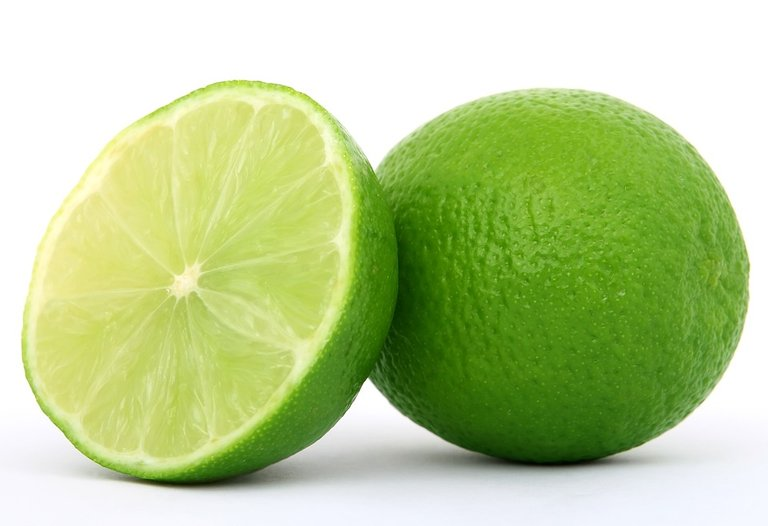Pixabay Image Source

## Basic Fractions Games

The basic fractions games presented here can be used for young ones as early as grade two or three. These games have visuals to allow for easy understanding.

Count Equal Parts

This Count Equal Parts game has the user guess how many equal parts they see. A keyboard is not needed for this game as you can click on the number you want for input.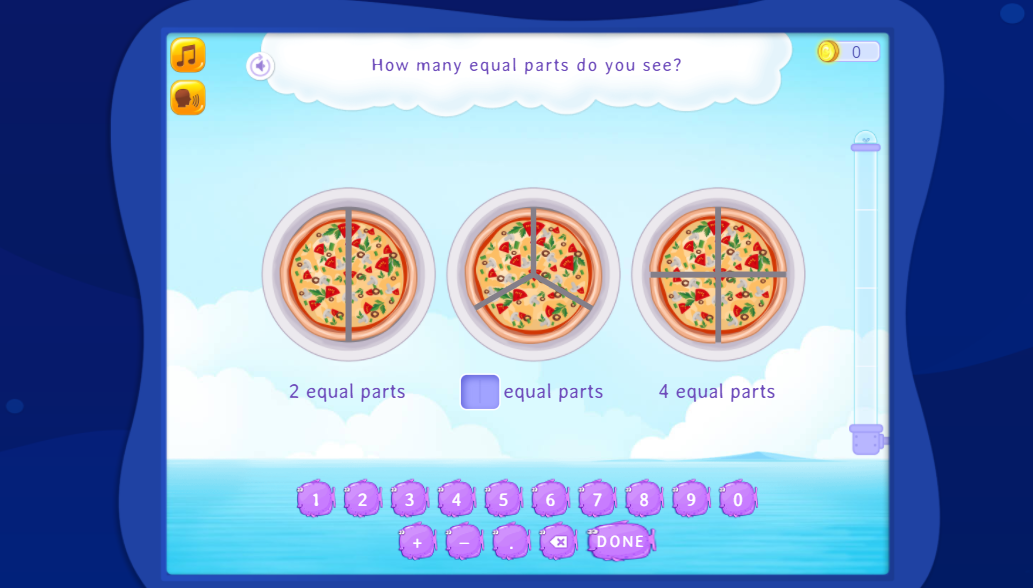Equal Parts

This Equal Parts game contains picture asking the user which ones have equal parts. When it comes to fractions it is important that the pieces are equal. The website categorizes this as a game for grade one. I would say this is good for grade two or three.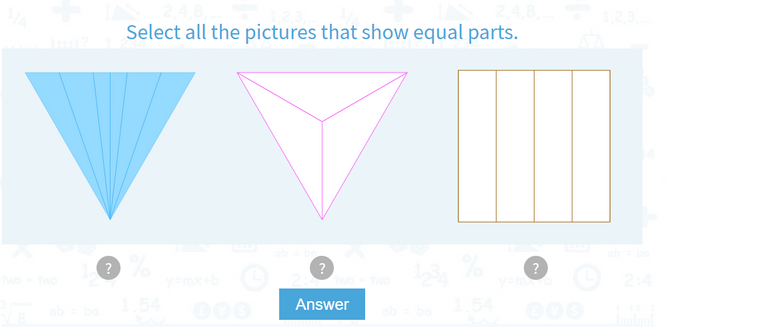Understand Unit Fractions

A unit fraction is a fraction that has a one in the numerator (top number of the fraction). It is one piece out of however many equal pieces.

This game has the user fill in the correct denominator (bottom number).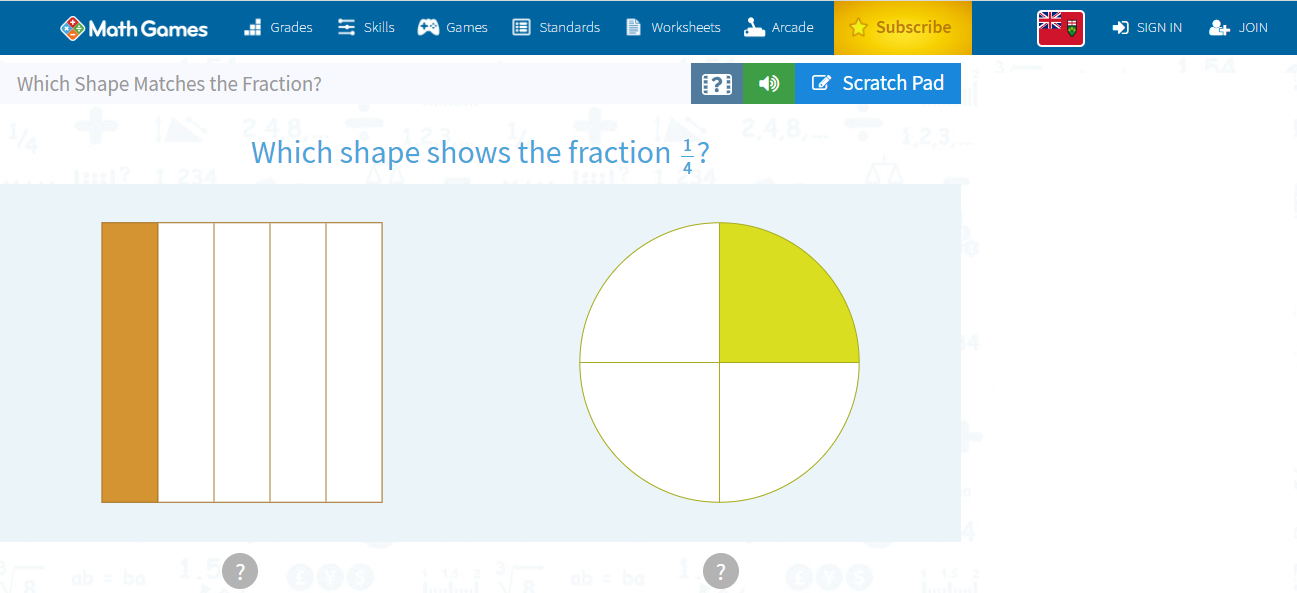Understand Non-Unit Fractions

The next step after understanding unit fractions is understanding non-unit fractions. The numerator of a fraction is not always one. This game helps with naming fractions based on the visuals provided in the game.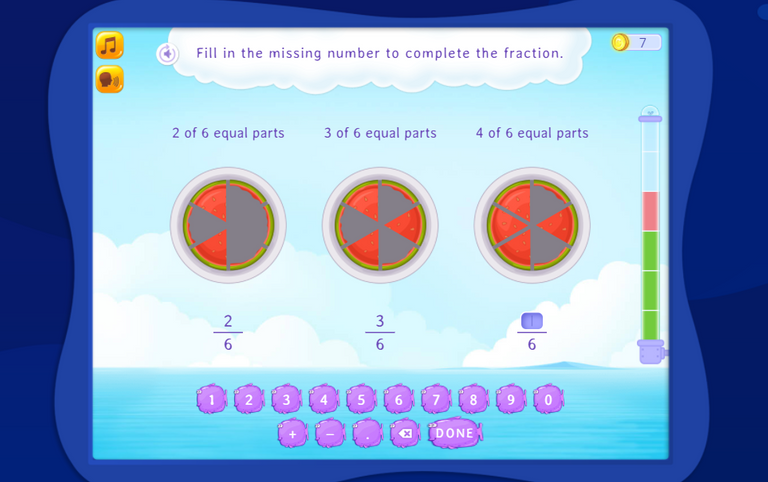Which Shape Matches The Fraction

This game is a matching game that has the user match a picture with the given fraction. The choices are in a this or that format.## Comparing Fractions

When it comes to comparing fractions, students have to comfortable with these signs `>, < and =`. The signs represent greater than, less than and equal to respectively.

Compare Fractions - Same Numerator or Denominator

This game has the user compare two fractions. The larger piece(s) represent more of the shaded region than the other.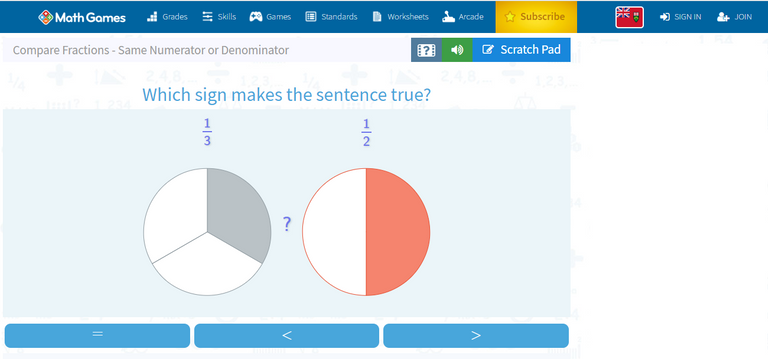Compare Fractions Using Real World Models

This game features food for comparing fractions. Choose <, > or = for comparing the slices that remain.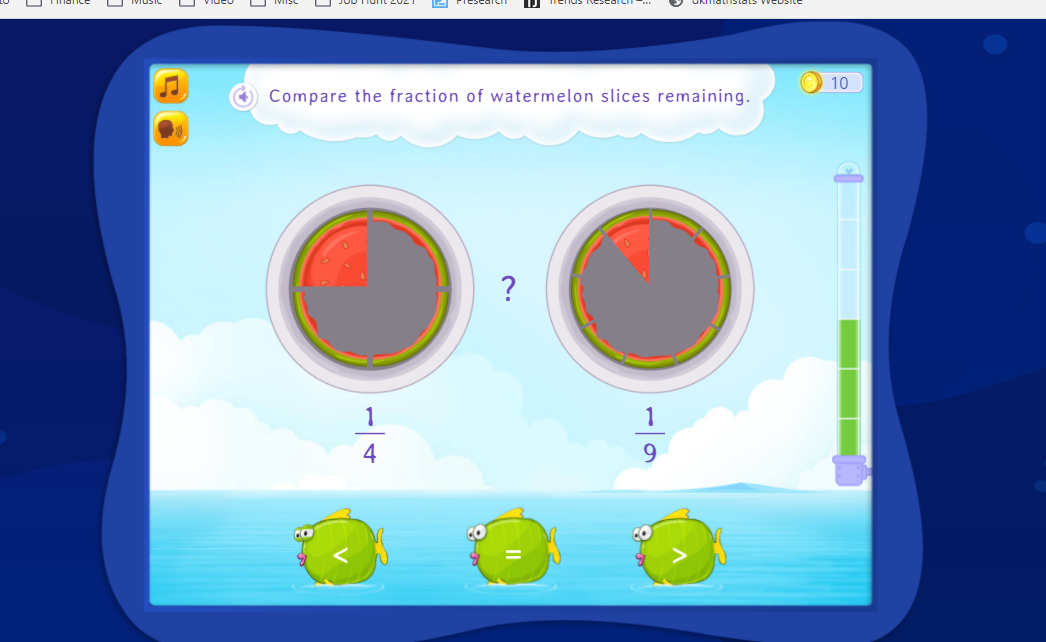Compare Fractions Using Fraction Strips

The visuals and the fractions strips in this game are helpful for those learning about comparing fractions. When choosing between two fractions, the larger fraction has more pieces being shaded. Choose between <, > or =.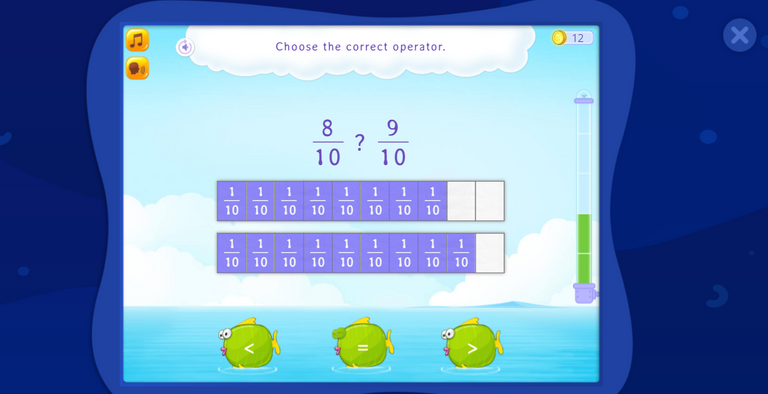Order Fractions With Same Denominator

With this fractions game, the player orders the fractions from greatest to least. As the denominator is the same, this game is not too hard.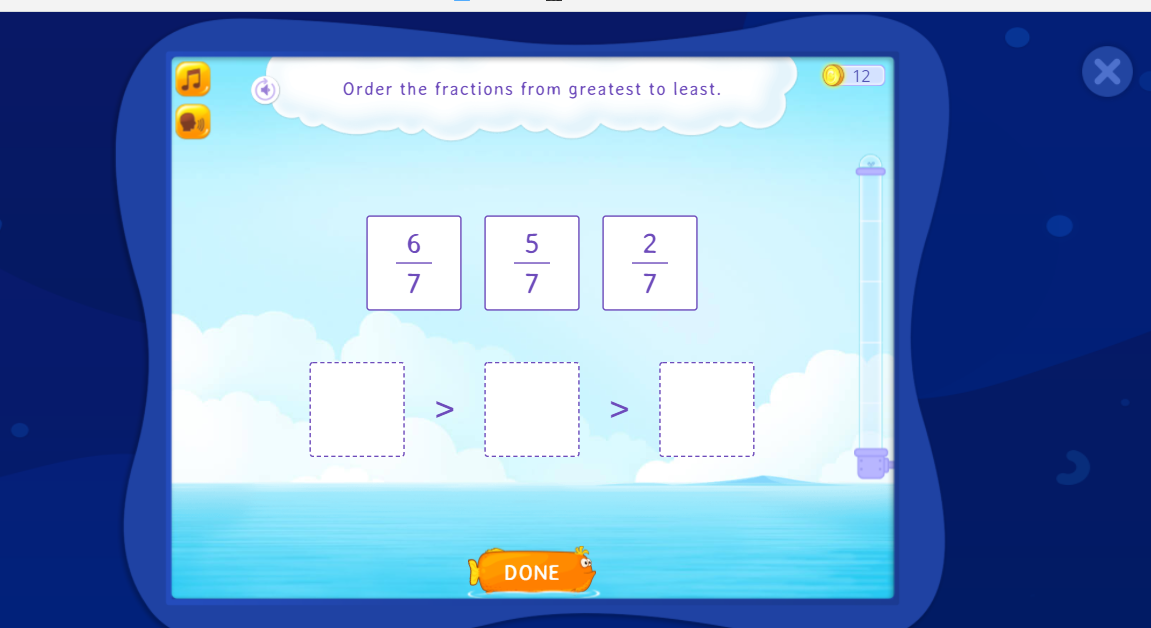Dirt Bike Comparing Fractions

In this comparing fractions game, the player chooses the correct symbol. The game is presented in a tug of war setting with flashcard style questions. Choose <, > or =. This game is not that easy as there is time pressure to win this tug of war game. There are no fraction strips or visuals provided in this game.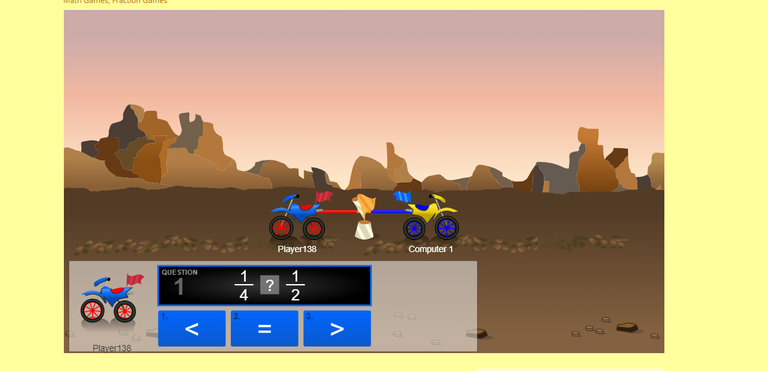## Mixed Numbers & Improper Fractions

A lot of fractions deal with part of one whole. The numeric value is between 0 and 1. For quantities in between whole numbers greater than one, we have mixed numbers and improper fractions.

Identify Mixed Numbers

This game focuses on identifying mixed number. The objective is to help the player understand that there is a whole number part and a fractional part in a mixed number.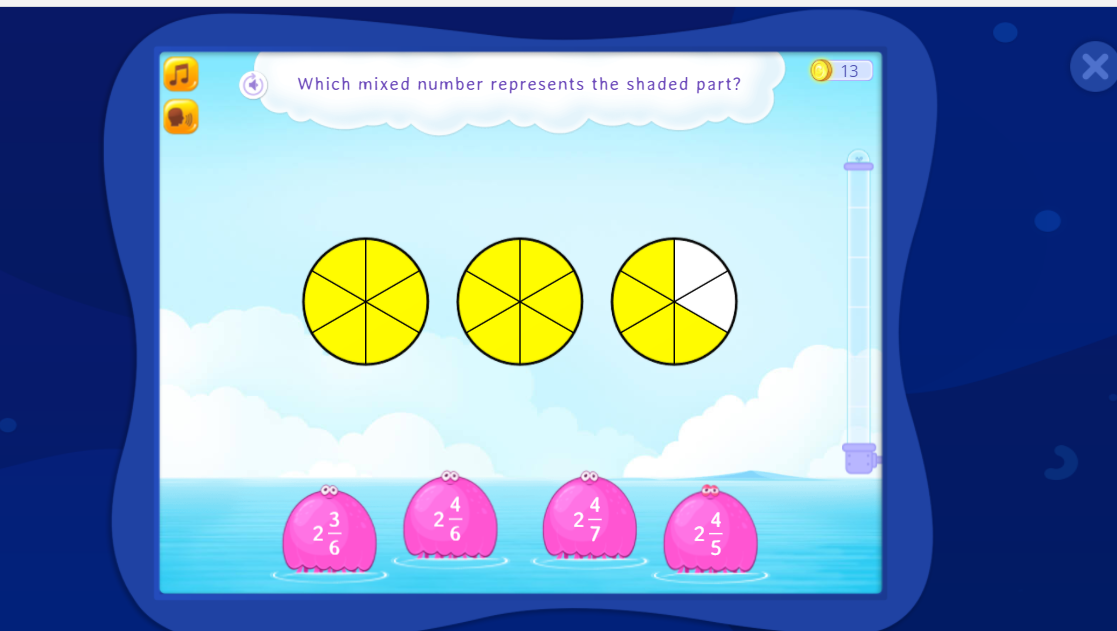Choose The Improper Fraction

In this game, players select the correct improper fraction that represents the shaded part. The improper fraction has the top number as the number of shaded pieces and the denominator represents the number of pieces in one whole.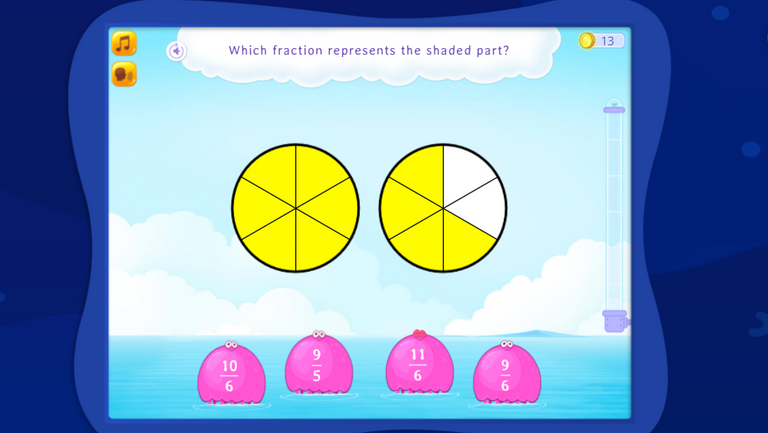Convert Between Improper Fractions and Mixed Numbers

This game contains no visuals compare to the previous two games. No visuals makes it harder. The task is to convert the improper fraction into a mixed number in simplest form.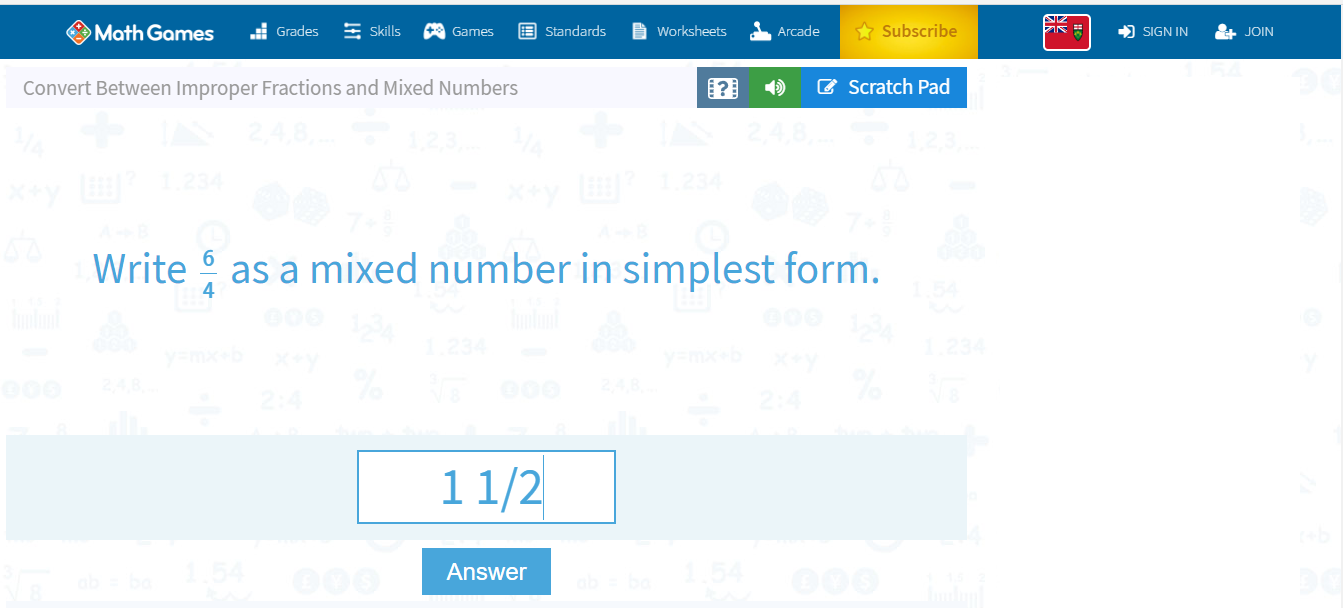## Equivalent Fractions

The concept of equivalent fractions can be tricky. Equivalent fractions are used when it comes to adding and subtracting fractions with common denominators.

Complete Equivalent Fractions

This game provides a good introduction to equivalent fractions as it has fraction strips. In the screenshot below, we multiply the top number and bottom number by 3 in one-third to obtain the answer of three-ninths.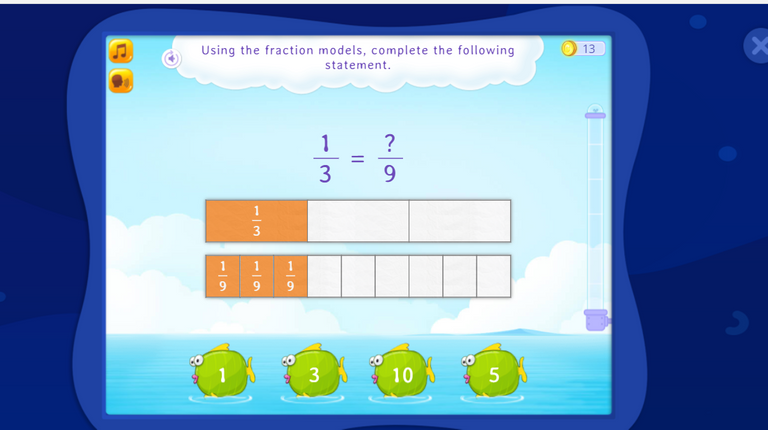Divide & Complete The Equivalent Fraction

Equivalent fractions are needed for reducing fractions to lowest terms. This game focuses on finding equivalent fractions through division.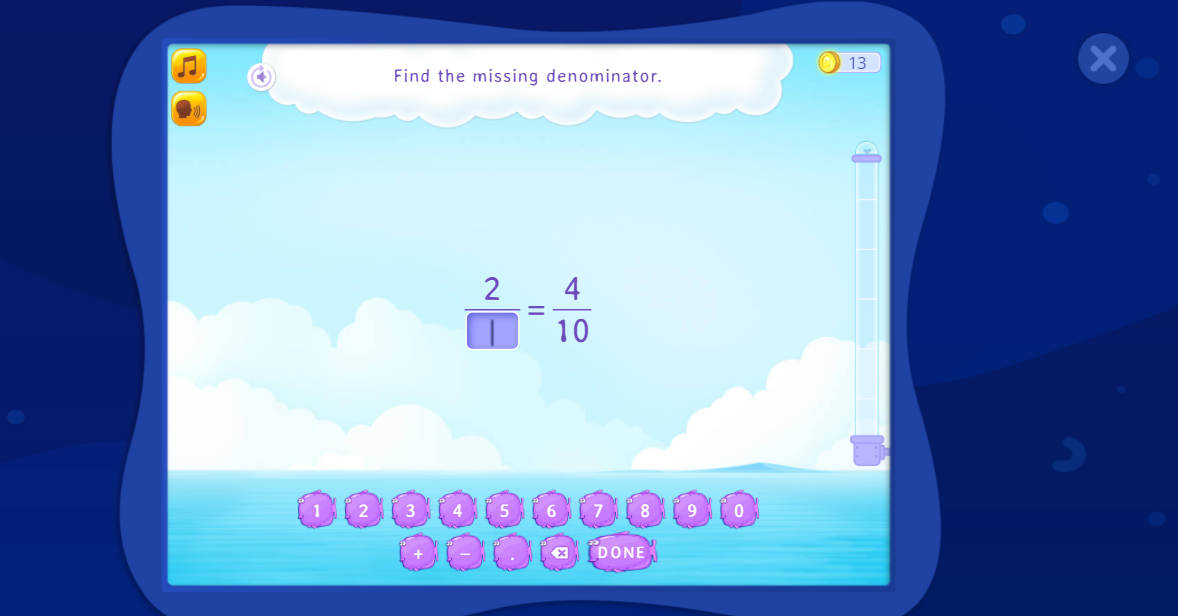Dirt Bike Proportions

This game from Arcademics.com is a racing game based on equivalent fractions. The player has to choose the correct value for `x` from the options below to complete the equivalent fraction. As this is a racing game, being able to find equivalent fractions is helpful but not that easy to do. I consider this game as a bit advanced.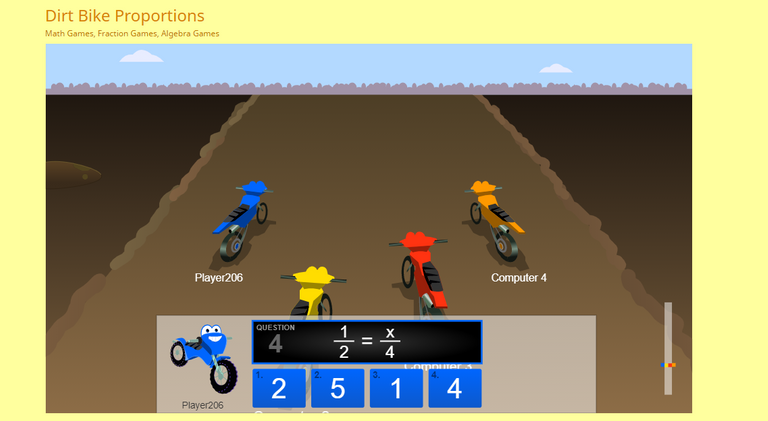## Adding & Subtracting Fractions

Adding & subtracting fractions is the next step after equivalent fractions. With me as a young student I do not recall using visuals. I wish I had some of these games when it came to learn fractions.

Add Fractions Using Models

This adding fractions game provides visuals in the form of fraction strips. You can visualize the equivalent fractions and see common denominators. Splashlearn has a subtracting fractions version too (see below).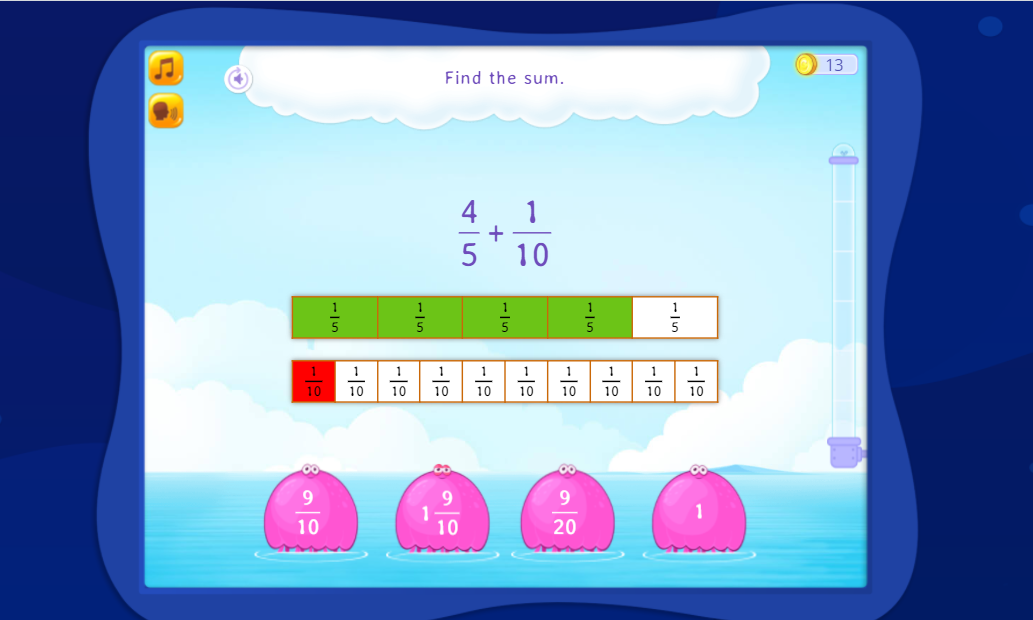Subtracting Fractions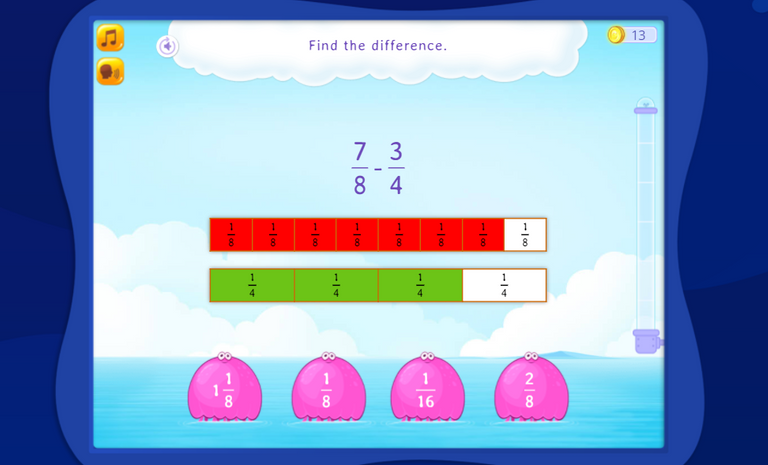Addition Game With Fractions

This game from Sheppard Software does not provide visuals. It is more for building accuracy and speed. They have multiple levels of difficulty. The hardest game setting would be Level 3b which is adding fractions with unlike denominators and then simplifying the answer.

You have the question that can be moved around with your mouse. Possible answers in the form of fruits are floating and moving around. Click on the correct answer to earn points in the game.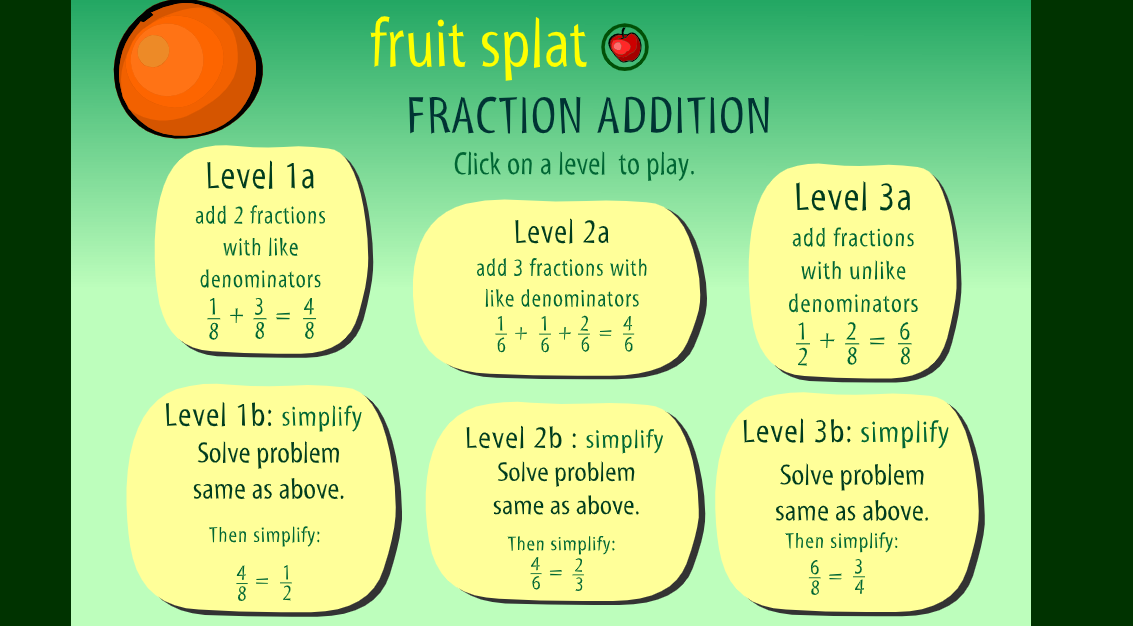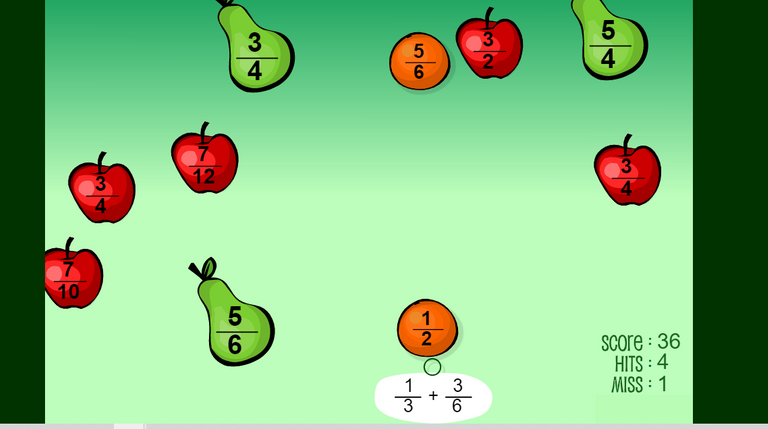## Multiplying & Dividing Fractions

There are also math games dedicated for multiplying and dividing fractions. Here are some of the games on this topic.

Choose The Multiplicaton Expression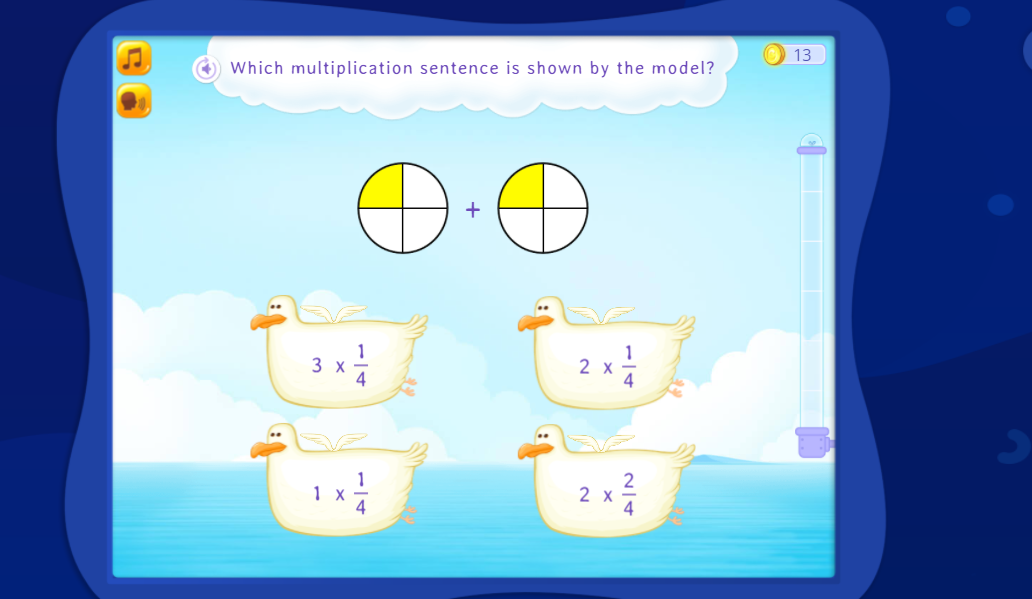Multiply Fractions Using Models

This game is nice as it provides visuals with different colours. In the screenshot below two-thirds times two-thirds gives four-ninths. This four-ninths is represented by the four squares in the top left corner. Another way to look at this is youu have two thirds and you take two-thirds of two-thirds. The keyword `of` is for multiplication.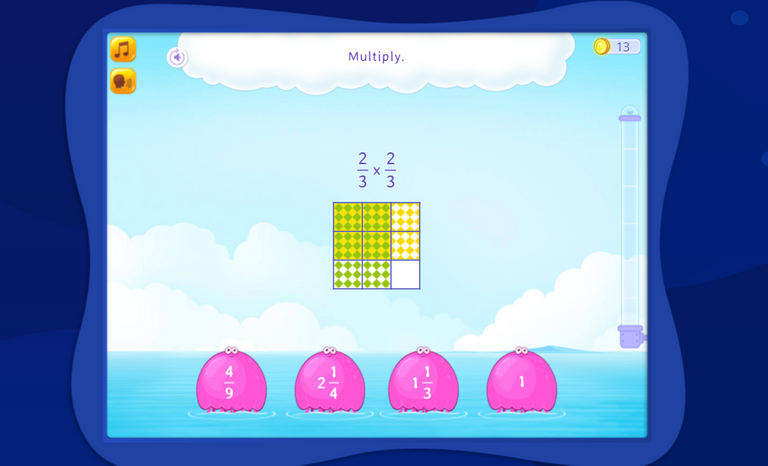Snow Sprint Multiplying Fractions

Arcademics.com has a game on multiplying fractions. No visuals are provided in this racing game. Get the correct answer in reduced form to give your character a boost towards the finish line.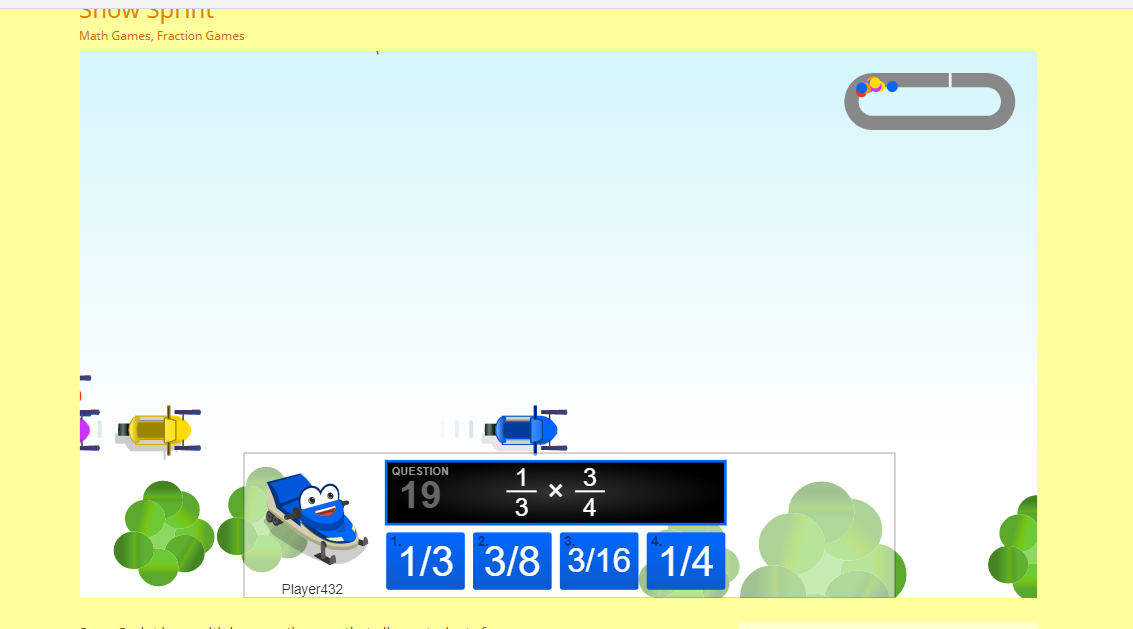Divide Unit Fraction By Whole Number

This game provides nice visuals in showing dividing a unit fraction by a whole number. The game is presents the questions in a multiple choice format.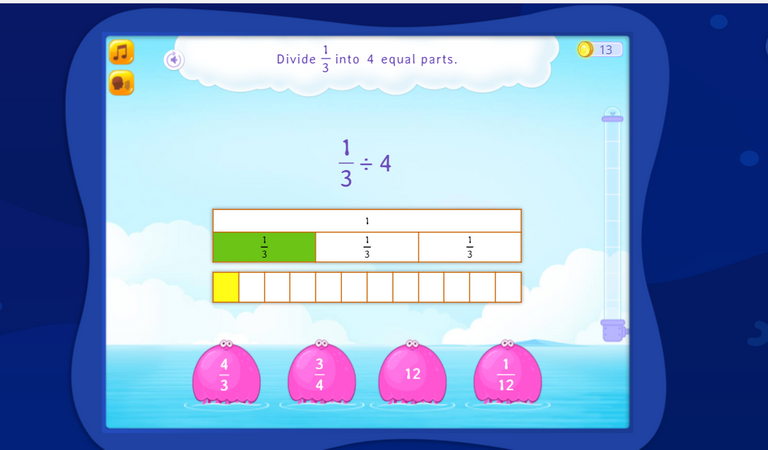## Other Fractions Games

I have some other fractions games that are not classified.

Simplifying Fractions Game

This simplifying fractions game is a nice game that helps with reducing fractions and building speed. The game features coffee which I think is not necessary but it provides a nice visual.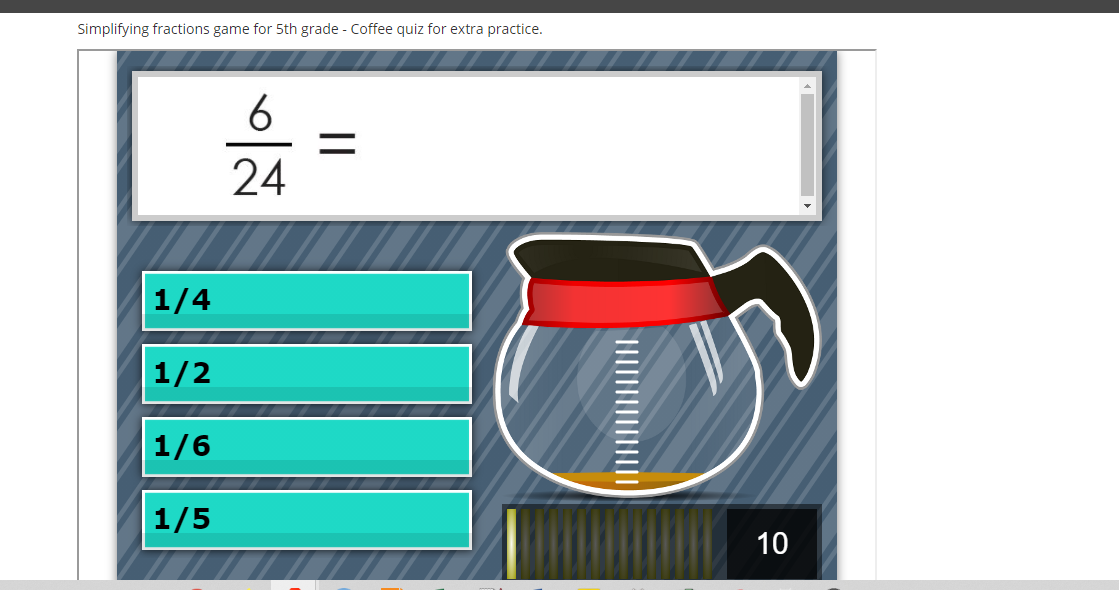Puppy Chase Decimals - Convert Fractions To Decimals

Puppy Chase Decimals is one of my favourite math games out there. You control your own puppy in a race. The questions are in a multiple choice format where you convert the given fraction to its corresponding decimal value.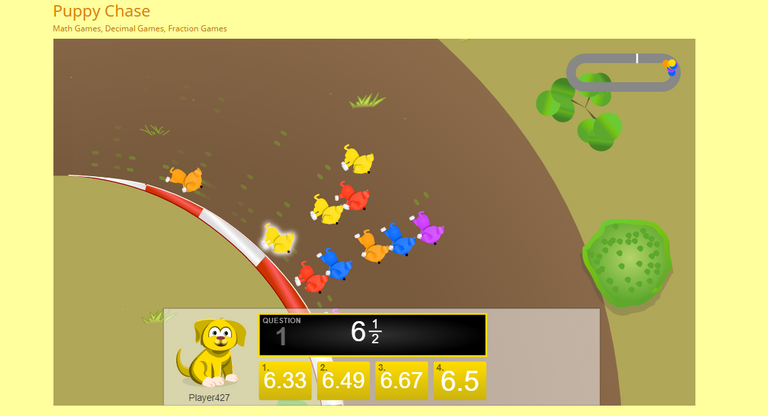Puzzle Pic Fractions

Puzzle Pic Fractions is a neat game which combines a puzzle and math. A fraction appears at the bottom. Drag the picture piece from the bottom right to the corresponding piece such that the number line red dot matches the given fraction.

As each game is random, you get a different picture/animal.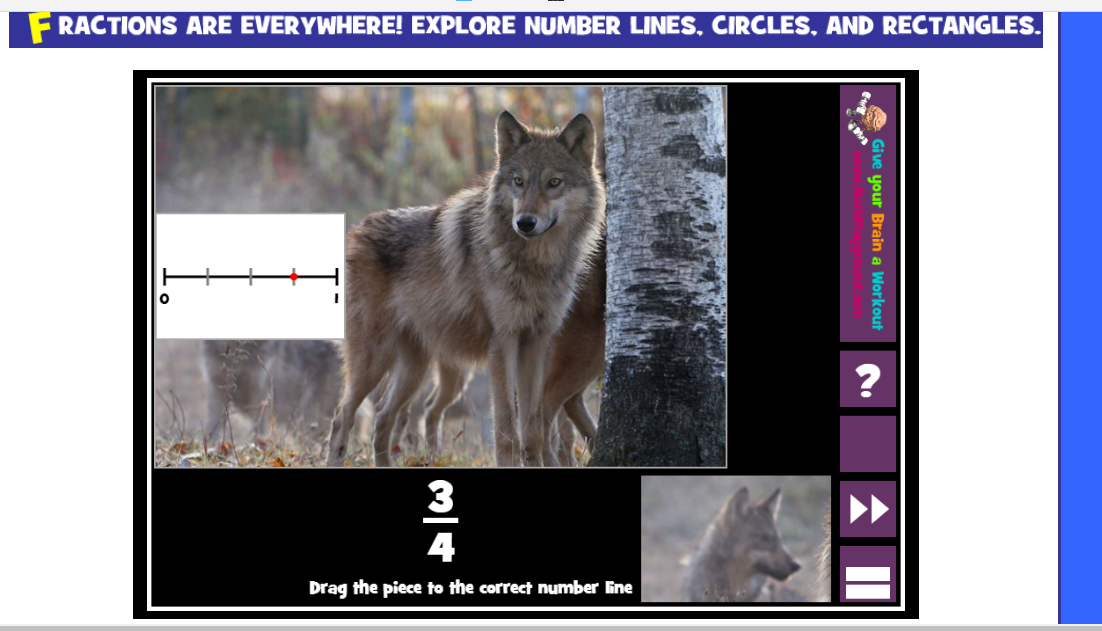## Splashlearn Website For Games

A lot of these fractions games are from the Splashlearn website. They have a very good collection of math games for fractions. They also have math games for other math topics.

There is no need to sign up for an account to play their games. On the website, it is free to sign up. This website is great as a supplementary resource or for homeschooling purposes.Pixabay Image Source - A Nice Image To End This Post

Thank you for reading.

Sort:

Muy buena la explicación y valioso el material.

Wow this has come up really well and is quite helpful for the students learning the portion of maths syllabus

The abundance of free online math games is very helpful for students, teachers and for homeschooling. I was wowed too in my research of fractions games.

This post is only a sample of what is out there with the games.